Documentation

### This is machine translation

Mouseover text to see original. Click the button below to return to the English version of the page.

# tfest

Transfer function estimation

## Syntax

```sys = tfest(data,np) sys = tfest(data,np,nz) sys = tfest(data,np,nz,iodelay) sys = tfest(___,Name,Value) sys = tfest(data,init_sys) sys = tfest(___,opt) ```

## Description

`sys = tfest(data,np)` estimates a continuous-time transfer function, `sys`, using time- or frequency-domain data, `data`, and contains `np` poles. The number of zeros in the `sys` is `max(np-1,0)`.

`sys = tfest(data,np,nz)` estimates a transfer function containing `nz` zeros.

`sys = tfest(data,np,nz,iodelay)` estimates a transfer function with transport delay for input/output pairs `iodelay`.

`sys = tfest(___,Name,Value)` uses additional options specified by one or more `Name,Value` pair arguments. All input arguments described for previous syntaxes also apply here.

`sys = tfest(data,init_sys)` uses the linear system `init_sys` to configure the initial parameterization of `sys`.

`sys = tfest(___,opt)` specifies the estimation behavior using the option set `opt`. All input arguments described for previous syntaxes also apply here.

## Input Arguments

 `data` Estimation data. For time domain estimation, `data` is an `iddata` object containing the input and output signal values. Time-series models, which are models that contain no measured inputs, cannot be estimated using `tfest`. Use `ar`, `arx` or `armax` for time-series models instead. For frequency domain estimation, `data` can be one of the following: `frd` or `idfrd` object that represents recorded frequency response data:Complex-values G(eiω) , for given frequencies ωAmplitude $|G|$ and phase shift $\phi =\mathrm{arg}G$ values`iddata` object with its properties specified as follows:`InputData` — Fourier transform of the input signal`OutputData` — Fourier transform of the output signal`Domain` — `'Frequency'` For multi-experiment data, the sample times and intersample behavior of all the experiments must match. `np` Number of poles in the estimated transfer function. `np` is a nonnegative number. For systems that are multiple-input, or multiple-output, or both: To use the same number of poles for all the input/output pairs, specify `np` as a scalar.To use different number of poles for the input/output pairs, specify `np` as an ny-by-nu matrix. ny is the number of outputs, and nu is the number of inputs. `nz` Number of zeros in the estimated transfer function. `nz` is a nonnegative number. For systems that are multiple-input, or multiple-output, or both: To use the same number of zeros for all the input/output pairs, specify `nz` as a scalar.To use a different number of zeros for the input/output pairs, specify `nz` as an Ny-by-Nu matrix. Ny is the number of outputs, and Nu is the number of inputs. For a continuous-time model, estimated using discrete-time data, set `nz <= np`. For discrete-time model estimation, specify `nz` as the number of zeros of the numerator polynomial of the transfer function. For example, `tfest(data,2,1,'Ts',data.Ts)` estimates a transfer function of the form ${b}_{1}{z}^{-1}/\left(1+{a}_{1}{z}^{-1}+{b}_{2}{z}^{-2}\right)$, while `tfest(data,2,2,'Ts',data.Ts)` estimates $\left({b}_{1}{z}^{-1}+{b}_{2}{z}^{-2}\right)/\left(1+{a}_{1}{z}^{-1}+{b}_{2}{z}^{-2}\right)$. Here z-1 is the Z-transform lag variable. For more information about discrete-time transfer functions, see Discrete-Time Representation. For an example, see Estimate Discrete-Time Transfer Function. `iodelay` Transport delay. For continuous-time systems, specify transport delays in the time unit stored in the `TimeUnit` property of `data`. For discrete-time systems, specify transport delays as integers denoting delay of a multiple of the sample time `Ts`. For a MIMO system with Ny outputs and Nu inputs, set `iodelay` to an Ny-by-Nu array. Each entry of this array is a numerical value that represents the transport delay for the corresponding input/output pair. You can also set `iodelay` to a scalar value to apply the same delay to all input/output pairs. The specified values are treated as fixed delays. `iodelay` must contain either nonnegative numbers or NaNs. Use NaN in the `iodelay` matrix to denote unknown transport delays. Use `[]` or `0` to indicate that there is no transport delay. `opt` Estimation options. `opt` is an options set, created using `tfestOptions`, that specifies estimation options including: Estimation objectiveHandling of initial conditionsNumerical search method to be used in estimation `init_sys` Linear system that configures the initial parameterization of `sys`. You obtain `init_sys` by either performing an estimation using measured data or by direct construction. If `init_sys` is an `idtf` model, `tfest` uses the parameters and constraints defined in `init_sys` as the initial guess for estimating `sys`. Use the `Structure` property of `init_sys` to configure initial guesses and constraints for the numerator, denominator, and transport lag. For example: To specify an initial guess for the numerator of `init_sys`, set `init_sys.Structure.Numerator.Value` to the initial guess.To specify constraints for the numerator of `init_sys`:Set `init_sys.Structure.Numerator.Minimum` to the minimum numerator coefficient valuesSet `init_sys.Structure.Numerator.Maximum` to the maximum numerator coefficient valuesSet `init_sys.Structure.Numerator.Free` to indicate which numerator coefficients are free for estimation If `init_sys` is not an `idtf` model, the software first converts `init_sys` to a transfer function. `tfest` uses the parameters of the resulting model as the initial guess for estimation. If `opt` is not specified, and `init_sys` was obtained by estimation, then the estimation options from `init_sys.Report.OptionsUsed` are used.

### Name-Value Pair Arguments

Specify optional comma-separated pairs of `Name,Value` arguments. `Name` is the argument name and `Value` is the corresponding value. `Name` must appear inside quotes. You can specify several name and value pair arguments in any order as `Name1,Value1,...,NameN,ValueN`.

 `'Ts'` Sample time. Use the following values for `Ts`: `0` — Continuous-time model.`data.Ts` — Discrete-time model. In this case, `np` and `nz` refer to the number of roots of `z^-1` for the numerator and denominator polynomials. Default: `0` `'InputDelay'` Input delay for each input channel, specified as a scalar value or numeric vector. For continuous-time systems, specify input delays in the time unit stored in the `TimeUnit` property. For discrete-time systems, specify input delays in integer multiples of the sample time `Ts`. For example, ```InputDelay = 3``` means a delay of three sample times. For a system with `Nu` inputs, set `InputDelay` to an `Nu`-by-1 vector. Each entry of this vector is a numerical value that represents the input delay for the corresponding input channel. You can also set `InputDelay` to a scalar value to apply the same delay to all channels. Default: 0 `'Feedthrough'` Feedthrough for discrete-time transfer function, specified as an Ny-by-Nu logical matrix. Ny is the number of outputs, and Nu is the number of inputs. To use the same feedthrough for all input-output channels, specify `Feedthrough` as a scalar. Consider a discrete-time model with two poles and three zeros: $H\left({z}^{-1}\right)=\frac{b0+b1{z}^{-1}+b2{z}^{-2}+b3{z}^{-3}}{1+a1{z}^{-1}+a2{z}^{-2}}$ When the model has direct feedthrough, `b0` is a free parameter whose value is estimated along with the rest of the model parameters `b1`, `b2`, `b3`, `a1`, `a2`. When the model has no feedthrough, `b0` is fixed to zero. For an example, see Estimate Discrete-Time Transfer Function With Feedthrough. Default: `false` (Ny,Nu)

## Output Arguments

`sys`

Identified transfer function, returned as an `idtf` model. This model is created using the specified model orders, delays and estimation options.

Information about the estimation results and options used is stored in the `Report` property of the model. `Report` has the following fields:

Report FieldDescription
`Status`

Summary of the model status, which indicates whether the model was created by construction or obtained by estimation.

`Method`

Estimation command used.

`InitializeMethod`

Algorithm used to initialize the numerator and denominator for estimation of continuous-time transfer functions using time-domain data, returned as one of the following values:

• `'iv'` — Instrument Variable approach.

• `'svf'` — State Variable Filters approach.

• `'gpmf'` — Generalized Poisson Moment Functions approach.

• `'n4sid'` — Subspace state-space estimation approach.

This field is especially useful to view the algorithm used when the `InitializeMethod` option in the estimation option set is `'all'`.

`N4Weight`

Weighting matrices used in the singular-value decomposition step when `InitializeMethod` is `'n4sid'`, returned as one of the following values:

• `'MOESP'` — Uses the MOESP algorithm by Verhaegen.

• `'CVA'` — Uses the canonical variable algorithm (CVA) by Larimore.

• `'SSARX'` — A subspace identification method that uses an ARX estimation based algorithm to compute the weighting.

This field is especially useful to view the weighting matrices used when the `N4Weight` option in the estimation option set is `'auto'`.

`N4Horizon`

Forward and backward prediction horizons used when `InitializeMethod` is `'n4sid'`, returned as a row vector with three elements — `[r sy su]`, where `r` is the maximum forward prediction horizon. `sy` is the number of past outputs, and `su` is the number of past inputs that are used for the predictions.

`InitialCondition`

Handling of initial conditions during model estimation, returned as one of the following values:

• `'zero'` — The initial conditions were set to zero.

• `'estimate'` — The initial conditions were treated as independent estimation parameters.

• `'backcast'` — The initial conditions were estimated using the best least squares fit.

This field is especially useful to view how the initial conditions were handled when the `InitialCondition` option in the estimation option set is `'auto'`.

`Fit`

Quantitative assessment of the estimation, returned as a structure. See Loss Function and Model Quality Metrics for more information on these quality metrics. The structure has the following fields:

FieldDescription
`FitPercent`

Normalized root mean squared error (NRMSE) measure of how well the response of the model fits the estimation data, expressed as a percentage.

`LossFcn`

Value of the loss function when the estimation completes.

`MSE`

Mean squared error (MSE) measure of how well the response of the model fits the estimation data.

`FPE`

Final prediction error for the model.

`AIC`

Raw Akaike Information Criteria (AIC) measure of model quality.

`AICc`

Small sample-size corrected AIC.

`nAIC`

Normalized AIC.

`BIC`

Bayesian Information Criteria (BIC).

`Parameters`

Estimated values of model parameters.

`OptionsUsed`

Option set used for estimation. If no custom options were configured, this is a set of default options. See `polyestOptions` for more information.

`RandState`

State of the random number stream at the start of estimation. Empty, `[]`, if randomization was not used during estimation. For more information, see `rng` in the MATLAB® documentation.

`DataUsed`

Attributes of the data used for estimation, returned as a structure with the following fields:

FieldDescription
`Name`

Name of the data set.

`Type`

Data type.

`Length`

Number of data samples.

`Ts`

Sample time.

`InterSample`

Input intersample behavior, returned as one of the following values:

• `'zoh'` — Zero-order hold maintains a piecewise-constant input signal between samples.

• `'foh'` — First-order hold maintains a piecewise-linear input signal between samples.

• `'bl'` — Band-limited behavior specifies that the continuous-time input signal has zero power above the Nyquist frequency.

`InputOffset`

Offset removed from time-domain input data during estimation. For nonlinear models, it is `[]`.

`OutputOffset`

Offset removed from time-domain output data during estimation. For nonlinear models, it is `[]`.

`Termination`

Termination conditions for the iterative search used for prediction error minimization. Structure with the following fields:

FieldDescription
`WhyStop`

Reason for terminating the numerical search.

`Iterations`

Number of search iterations performed by the estimation algorithm.

`FirstOrderOptimality`

$\infty$-norm of the gradient search vector when the search algorithm terminates.

`FcnCount`

Number of times the objective function was called.

`UpdateNorm`

Norm of the gradient search vector in the last iteration. Omitted when the search method is `'lsqnonlin'` or `'fmincon'`.

`LastImprovement`

Criterion improvement in the last iteration, expressed as a percentage. Omitted when the search method is `'lsqnonlin'` or `'fmincon'`.

`Algorithm`

Algorithm used by `'lsqnonlin'` or `'fmincon'` search method. Omitted when other search methods are used.

For estimation methods that do not require numerical search optimization, the `Termination` field is omitted.

For more information on using `Report`, see Estimation Report.

## Examples

collapse all

Load time-domain system response data and use it to estimate a transfer function for the system.

```load iddata1 z1; np = 2; sys = tfest(z1,np);```

`z1` is an `iddata` object that contains time-domain, input-output data.

`np` specifies the number of poles in the estimated transfer function.

`sys` is an `idtf` model containing the estimated transfer function.

To see the numerator and denominator coefficients of the resulting estimated model `sys`, enter:

`sys.Numerator`
```ans = 1×2 2.4554 176.9856 ```
`sys.Denominator`
```ans = 1×3 1.0000 3.1625 23.1631 ```

To view the uncertainty in the estimates of the numerator and denominator and other information, use `tfdata`.

Load time-domain system response data and use it to estimate a transfer function for the system.

```load iddata2 z2; np = 2; nz = 1; sys = tfest(z2,np,nz);```

`z2` is an `iddata` object that contains time-domain system response data.

`np` and `nz` specify the number of poles and zeros in the estimated transfer function, respectively.

`sys` is an `idtf` model containing the estimated transfer function.

Load time-domain system response data and use it to estimate a transfer function for the system. Specify a known transport delay for the transfer function.

```load iddata2 z2; np = 2; nz = 1; iodelay = 0.2; sys = tfest(z2,np,nz,iodelay);```

`z2` is an `iddata` object that contains time-domain system response data.

`np` and `nz` specify the number of poles and zeros in the estimated transfer function, respectively.

`iodelay` specifies the transport delay for the estimated transfer function as 0.2 seconds.

`sys` is an `idtf` model containing the estimated transfer function, with `IODelay` property set to 0.2 seconds.

Load time-domain system response data and use it to estimate a transfer function for the system. Specify an unknown transport delay for the transfer function.

```load iddata2 z2; np = 2; nz = 1; iodelay = NaN; sys = tfest(z2,np,nz,iodelay);```

`z2` is an `iddata` object that contains time-domain system response data.

`np` and `nz` specify the number of poles and zeros in the estimated transfer function, respectively.

`iodelay` specifies the transport delay for the estimated transfer function. `iodelay = NaN` denotes the transport delay as an unknown parameter to be estimated.

`sys` is an `idtf` model containing the estimated transfer function, whose `IODelay` property is estimated using data.

`load iddata2 z2;`

`z2` is an `iddata` object that contains the time-domain system response data.

Estimate a discrete-time transfer function with two poles and one zero. Specify the sample time as 0.1 sec and the transport delay as 2 sec.

```np = 2; nz = 1; iodelay = 2; Ts = 0.1; sysd = tfest(z2,np,nz,iodelay,'Ts',Ts)```
```sysd = From input "u1" to output "y1": 1.8 z^-1 z^(-2) * ---------------------------- 1 - 1.418 z^-1 + 0.6613 z^-2 Sample time: 0.1 seconds Discrete-time identified transfer function. Parameterization: Number of poles: 2 Number of zeros: 1 Number of free coefficients: 3 Use "tfdata", "getpvec", "getcov" for parameters and their uncertainties. Status: Estimated using TFEST on time domain data "z2". Fit to estimation data: 80.26% FPE: 2.095, MSE: 2.063 ```

By default, the model has no feedthrough, and the numerator polynomial of the estimated transfer function has a zero leading coefficient `b0`. To estimate `b0`, specify the `Feedthrough` property during estimation.

`load iddata5 z5`

First estimate a discrete-time transfer function model with two poles, one zero, and no feedthrough.

```np = 2; nz = 1; model = tfest(z5,np,nz,'Ts',z5.Ts);```

The estimated transfer function has the form:

`$H\left({z}^{-1}\right)=\frac{b1{z}^{-1}+b2{z}^{-2}}{1+a1{z}^{-1}+a2{z}^{-2}}$`

By default, the model has no feedthrough, and the numerator polynomial of the estimated transfer function has a zero leading coefficient `b0`. To estimate `b0`, specify the `Feedthrough` property during estimation.

`model = tfest(z5,np,nz,'Ts',z5.Ts,'Feedthrough',true);`

The numerator polynomial of the estimated transfer function now has a nonzero leading coefficient:

`$H\left({z}^{-1}\right)=\frac{b0+b1{z}^{-1}+b2{z}^{-2}}{1+a1{z}^{-1}+a2{z}^{-2}}$`

Compare two discrete-time models with and without feedthrough and transport delay.

If there is a delay from the measured input to output, it can be attributed to a lack of feedthrough or to a true transport delay. For discrete-time models, absence of feedthrough corresponds to a lag of 1 sample between the input and output. Estimating a model with `Feedthrough = false` and `IODelay = 0` thus produces a discrete-time system that is equivalent to a system with `Feedthrough = true` and `IODelay = 1`. Both systems show the same time- and frequency-domain responses, for example, on step and Bode plots. However, you get different results if you reduce these models using `balred` or convert them to their continuous-time representation. Therefore, you should check if the observed delay should be attributed to transport delay or to a lack of feedthrough.

Estimate a discrete-time model with no feedthrough.

```load iddata1 z1 np = 2; nz = 2; model1 = tfest(z1,np,nz,'Ts',z1.Ts);```

`model1` has a transport delay of 1 sample and its `IODelay` property is 0. Its numerator polynomial begins with ${z}^{-1}$.

Estimate another discrete-time model with feedthrough and 1 sample input-output delay.

`model2 = tfest(z1,np,nz-1,1,'Ts',z1.Ts,'Feedthrough',true);`

Compare the Bode response of the models.

`bode(model1,model2);`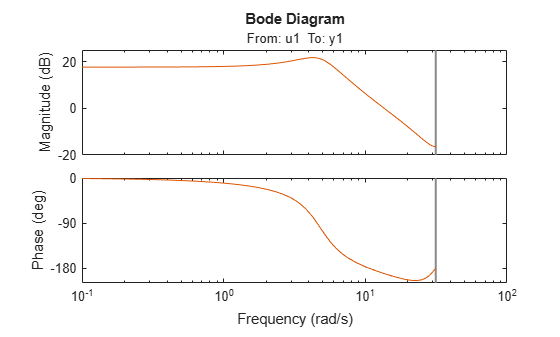The equations for `model1` and `model2` are equivalent, but the transport delay of `model2` has been absorbed into the numerator of `model1`.

Convert the models to continuous time, and compare their Bode responses.

`bode(d2c(model1),d2c(model2));`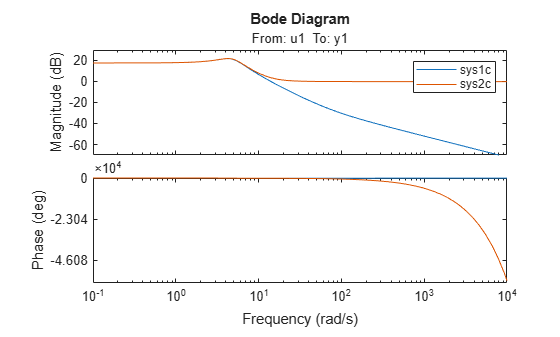As the plot shows, the Bode responses of the two models do not match when you convert them to continuous time.

Estimate a 2-input, 1-output discrete-time transfer function with a delay of 2 samples on first input and zero seconds on the second input. Both inputs have no feedthrough.

Split data into estimation and validation data sets.

```load iddata7 z7 ze = z7(1:300); zv = z7(200:400);```

Estimate a 2-input, 1-output transfer function with 2 poles and 1 zero for each input-to-output transfer function.

```Lag = [2;0]; Ft = [false,false]; model = tfest(ze,2,1,'Ts',z7.Ts,'Feedthrough',Ft,'InputDelay',Lag);```

Choice of `Feedthrough` dictates whether the leading numerator coefficient is zero (no feedthrough) or not (nonzero feedthrough). Delays are expressed separately using `InputDelay` or `IODelay` property. This example uses `InputDelay` to express the delays.

Validate the estimated model. Exclude the data outliers for validation.

```I = 1:201; I(114:118) = []; opt = compareOptions('Samples',I); compare(zv,model,opt)```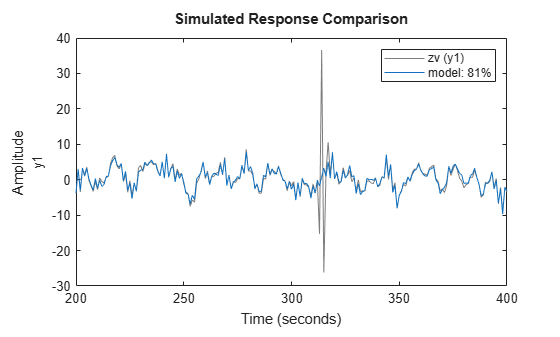Identify a 15th order transfer function model by using regularized impulse response estimation

`load regularizationExampleData m0simdata;`

Obtain regularized impulse response (FIR) model.

```opt = impulseestOptions('RegularizationKernel','DC'); m0 = impulseest(m0simdata,70,opt);```

Convert model into a transfer function model after reducing order to 15.

`m = idtf(balred(idss(m0),15));`

Compare the model output with data.

`compare(m0simdata,m);`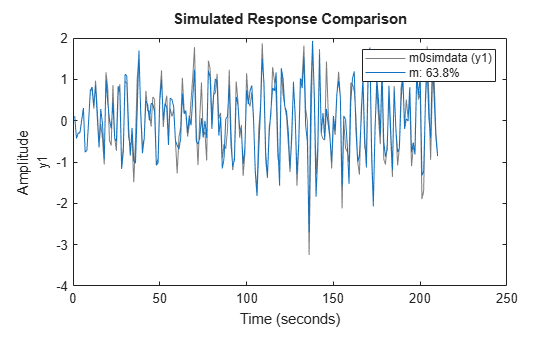Create the option set for `tfest`.

`opt = tfestOptions('InitializeMethod','n4sid','Display','on','SearchMethod','lsqnonlin');`

`opt` specifies that the initialization method as `'n4sid'`, and the search method as `'lsqnonlin'`. It also specifies that the loss-function values for each iteration be shown.

Load time-domain system response data and use it to estimate a transfer function for the system. Specify the estimation options using `opt`.

```load iddata2 z2; np = 2; nz = 1; iodelay = 0.2; sysc = tfest(z2,np,nz,iodelay,opt);```

`z2` is an `iddata` object that contains time-domain system response data.

`np` and `nz` specify the number of poles and zeros in the estimated transfer function, respectively.

`iodelay` specifies the transport delay for the estimated transfer function as 0.2 seconds.

`opt` specifies the estimation options.

`sys` is an `idtf` model containing the estimated transfer function.

Load time-domain system response data, and use it to estimate a transfer function for the system. Specify the input delay for the estimated transfer function.

```load iddata2 z2; np = 2; nz = 1; input_delay = 0.2; sys = tfest(z2,np,nz,'InputDelay',input_delay);```

`z2` is an `iddata` object that contains time-domain system response data.

`np` and `nz` specify the number of poles and zeros in the estimated transfer function, respectively.

`input_delay` specifies the input delay for the estimated transfer function as 0.2 seconds.

`sys` is an `idtf` model containing the estimated transfer function with an input delay of 0.2 seconds.

This example requires a Control System Toolbox™ license.

Obtain frequency-response data.

For example, use `bode` to obtain the magnitude and phase response data for the following system:

`$H\left(s\right)=\frac{s+0.2}{{s}^{3}+2{s}^{2}+s+1}$`

Use 100 frequency points, ranging from 0.1 rad/s to 10 rad/s, to obtain the frequency-response data. Use `frd` to create a frequency-response data object.

```freq = logspace(-1,1,100); [mag,phase] = bode(tf([1 0.2],[1 2 1 1]),freq); data = frd(mag.*exp(1j*phase*pi/180),freq);```

Estimate a transfer function using `data`.

```np = 3; nz = 1; sys = tfest(data,np,nz);```

`np` and `nz` specify the number of poles and zeros in the estimated transfer function, respectively.

`sys` is an `idtf` model containing the estimated transfer function.

```load co2data; Ts = 0.5; data = iddata(Output_exp1,Input_exp1,Ts);```

`data` is an `iddata` object and has a sample rate of 0.5 seconds.

Specify the search method as `gna`. Also specify the maximum search iterations and input/output offsets.

```opt = tfestOptions('SearchMethod','gna'); opt.InputOffset = [170;50]; opt.OutputOffset = mean(data.y(1:75)); opt.SearchOptions.MaxIterations = 50;```

`opt` is an estimation option set that specifies the search method as `gna`, with a maximum of 50 iterations. `opt` also specifies the input offset and the output offset.

Estimate a transfer function using the measured data and the estimation option set. Specify the transport delays from the inputs to the output.

```np = 3; nz = 1; iodelay = [2 5]; sys = tfest(data,np,nz,iodelay,opt);```

`iodelay` specifies the input to output delay from the first and second inputs to the output as 2 seconds and 5 seconds, respectively.

`sys` is an `idtf` model containing the estimated transfer function.

Load time-domain system response data and use it to estimate a transfer function for the system. Specify the known and unknown transport delays.

```load co2data; Ts = 0.5; data = iddata(Output_exp1,Input_exp1,Ts);```

`data` is an `iddata` object and has a sample rate of 0.5 seconds.

Specify the search method as `gna`. Also specify the maximum search iterations and input/output offsets.

```opt = tfestOptions('Display','on','SearchMethod','gna'); opt.InputOffset = [170; 50]; opt.OutputOffset = mean(data.y(1:75)); opt.SearchOptions.MaxIterations = 50;```

`opt` is an estimation option set that specifies the search method as `gna`, with a maximum of 50 iterations. `opt` also specifies the input/output offsets.

Estimate the transfer function. Specify the unknown and known transport delays.

```np = 3; nz = 1; iodelay = [2 nan]; sys = tfest(data,np,nz,iodelay,opt);```

`iodelay` specifies the transport delay from the first input to the output as 2 seconds. Using `NaN` specifies the transport delay from the second input to the output as unknown.

`sys` is an `idtf` model containing the estimated transfer function.

Create a transfer function model with the expected numerator and denominator structure and delay constraints.

In this example, the experiment data consists of two inputs and one output. Both transport delays are unknown and have an identical upper bound. Additionally, the transfer functions from both inputs to the output are identical in structure.

```init_sys = idtf(NaN(1,2),[1,NaN(1,3)],'IODelay',NaN); init_sys.Structure(1).IODelay.Free = true; init_sys.Structure(1).IODelay.Maximum = 7;```

`init_sys` is an `idtf` model describing the structure of the transfer function from one input to the output. The transfer function consists of one zero, three poles and a transport delay. The use of `NaN` indicates unknown coefficients.

`init_sys.Structure(1).IODelay.Free = true` indicates that the transport delay is not fixed.

`init_sys.Structure(1).IODelay.Maximum = 7` sets the upper bound for the transport delay to 7 seconds.

Specify the transfer function from both inputs to the output.

`init_sys = [init_sys,init_sys];`

Load time-domain system response data and use it to estimate a transfer function.

```load co2data; Ts = 0.5; data = iddata(Output_exp1,Input_exp1,Ts); opt = tfestOptions('Display','on','SearchMethod','gna'); opt.InputOffset = [170;50]; opt.OutputOffset = mean(data.y(1:75)); opt.SearchOptions.MaxIterations = 50; sys = tfest(data,init_sys,opt);```

`data` is an `iddata` object and has a sample rate of 0.5 seconds.

`opt` is an estimation option set that specifies the search method as `gna`, with a maximum of 50 iterations. `opt` also specifies the input offset and the output offset.

`sys` is an `idtf` model containing the estimated transfer function.

Analyze the estimation result by comparison.

```opt2 = compareOptions; opt2.InputOffset = opt.InputOffset; opt2.OutputOffset = opt.OutputOffset; compare(data,sys,opt2)```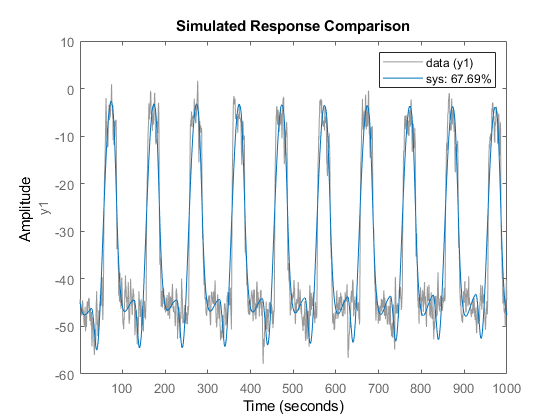Estimate a multiple-input, single-output transfer function containing different number of poles for input/output pairs for given data.

This example requires a Control System Toolbox™ license.

Obtain frequency-response data.

For example, use `frd` to create a frequency-response data model for the following system:

`$G=\left[\begin{array}{c}{e}^{-4s}\frac{s+2}{{s}^{3}+2{s}^{2}+4s+5}\\ {e}^{-0.6s}\frac{5}{{s}^{4}+2{s}^{3}+{s}^{2}+s}\end{array}\right]$`

Use 100 frequency points, ranging from 0.01 rad/s to 100 rad/s, to obtain the frequency-response data.

```G = tf({[1 2],},{[1 2 4 5],[1 2 1 1 0]},0,'IODelay',[4 0.6]); data = frd(G,logspace(-2,2,100));```

`data` is an `frd` object containing the continuous-time frequency response for `G`.

Estimate a transfer function for `data`.

``` np = [3 4]; nz = [1 0]; iodelay = [4 0.6]; sys = tfest(data,np,nz,iodelay);```

`np` specifies the number of poles in the estimated transfer function. The first element of `np` indicates that the transfer function from the first input to the output contains 3 poles. Similarly, the second element of `np` indicates that the transfer function from the second input to the output contains 4 poles.

`nz` specifies the number of zeros in the estimated transfer function. The first element of `nz` indicates that the transfer function from the first input to the output contains 1 zero. Similarly, the second element of `np` indicates that the transfer function from the second input to the output does not contain any zeros.

`iodelay` specifies the transport delay from the first input to the output as 4 seconds. The transport delay from the second input to the output is specified as 0.6 seconds.

`sys` is an `idtf` model containing the estimated transfer function.

Estimate a transfer function describing an unstable system for given data.

Obtain frequency-response data.

For example, use `frd` to create frequency-response data model for the following system:

`$G=\left[\begin{array}{c}\frac{s+2}{{s}^{3}+2{s}^{2}+4s+5}\\ \frac{5}{{s}^{4}+2{s}^{3}+{s}^{2}+s+1}\end{array}\right]$`

Use 100 frequency points, ranging from 0.01 rad/s to 100 rad/s, to obtain the frequency-response data.

```G = idtf({[1 2], 5},{[1 2 4 5],[1 2 1 1 1]}); data = idfrd(G,logspace(-2,2,100));```

`data` is an `idfrd` object containing the continuous-time frequency response for `G`.

Estimate a transfer function for `data`.

```np = [3 4]; nz = [1 0]; sys = tfest(data,np,nz);```

`np` specifies the number of poles in the estimated transfer function. The first element of `np` indicates that the transfer function from the first input to the output contains 3 poles. Similarly, the second element of `np` indicates that the transfer function from the second input to the output contains 4 poles.

`nz` specifies the number of zeros in the estimated transfer function. The first element of `nz` indicates that the transfer function from the first input to the output contains 1 zero. Similarly, the second element of `nz` indicates that the transfer function from the second input to the output does not contain any zeros.

`sys` is an `idtf` model containing the estimated transfer function.

`pole(sys)`
```ans = 7×1 complex -1.5260 + 0.0000i -0.2370 + 1.7946i -0.2370 - 1.7946i -1.4656 + 0.0000i -1.0000 + 0.0000i 0.2328 + 0.7926i 0.2328 - 0.7926i ```

`sys` is an unstable system as verified by the pole display.

Load the high density frequency response measurement data. The data corresponds to an unstable process maintained at equilibrium using feedback control.

`load HighModalDensityData FRF f`

Package the data as an `idfrd` object for identification and, find the Bode magnitude response.

```G = idfrd(permute(FRF,[2 3 1]),f,0,'FrequencyUnit','Hz'); bodemag(G)```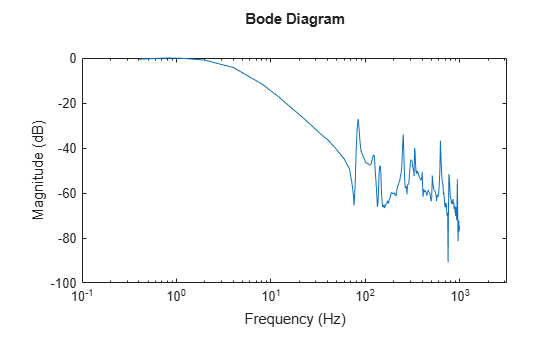Estimate a transfer function with 32 poles and 32 zeros, and compare the Bode magnitude response.

```sys = tfest(G,32,32); bodemag(G, sys) xlim([0.01,2e3]) legend```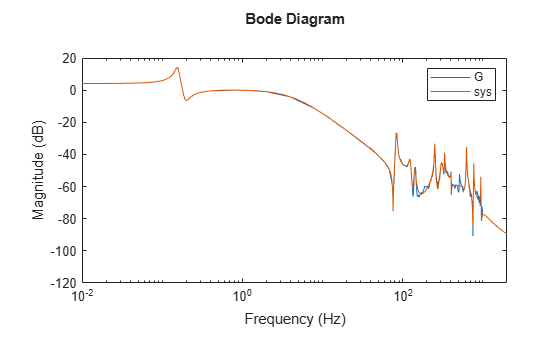collapse all

### Compatibility

Starting in R2016b, a new algorithm is used for performing transfer function estimation from frequency-domain data. You are likely to see faster and more accurate results with the new algorithm, particularly for data with dynamics over a large range of frequencies and amplitudes. However, the estimation results may not match results from previous releases. To perform estimation using the previous estimation algorithm, append `'-R2016a'` to the syntax.

For example, suppose that you are estimating a transfer function model with `np` poles using frequency-domain data, `data`.

`sys = tfest(data,np)`

To use the previous estimation algorithm, use the following syntax.

`sys = tfest(data,np,'-R2016a')`

## Algorithms

collapse all

The details of the estimation algorithms used by `tfest` vary depending on a variety of factors, including the sampling of the estimated model and the estimation data.

### Continuous-Time Transfer Function Estimation Using Time-Domain Data

#### Parameter Initialization

The estimation algorithm initializes the estimable parameters using the method specified by the `InitializeMethod` estimation option. The default method is the Instrument Variable (IV) method.

The State-Variable Filters (SVF) approach and the Generalized Poisson Moment Functions (GPMF) approach to continuous-time parameter estimation use prefiltered data  . The constant $\frac{1}{\lambda }$ in  and  corresponds to the initialization option (`InitializeOptions`) field `FilterTimeConstant`. IV is the simplified refined IV method and is called SRIVC in . This method has a prefilter that is the denominator of the current model, initialized with SVF. This prefilter is iterated up to `MaxIterations` times, until the model change is less than `Tolerance`. `MaxIterations` and `Tolerance` are options that you can specify using the `InitializeOptions` structure. The `'n4sid'` initialization option estimates a discrete-time model, using the N4SID estimation algorithm, that it transforms to continuous-time using `d2c`.

You use `tfestOptions` to create the option set used to estimate a transfer function.

#### Parameter Update

The initialized parameters are updated using a nonlinear least-squares search method, specified by the `SearchMethod` estimation option. The objective of the search method is to minimize the weighted prediction error norm.

### Discrete-Time Transfer Function Estimation Using Time-Domain Data

For discrete-time data, `tfest` uses the same algorithm as `oe` to determine the numerator and denominator polynomial coefficients. In this algorithm, the initialization is performed using `arx`, followed by nonlinear least-squares search based updates to minimize a weighted prediction error norm.

### Continuous-Time Transfer Function Estimation Using Continuous-Time Frequency-Domain Data

The estimation algorithm performs the following tasks:

1. Perform a bilinear mapping to transform the domain (frequency grid) of the transfer function. For continuous-time models, the imaginary axis is transformed to the unit disk. For discrete-time models, the original domain unit disk is transformed to another unit disk.

2. Perform S-K iterations  to solve a nonlinear least-squares problem — Consider a multi-input single-output system. The nonlinear least-squares problem is to minimize the loss function:

`$\underset{D,{N}_{i}}{\text{minimize}}\sum _{k=1}^{{n}_{f}}{|W\left({\omega }_{k}\right)\left(y\left({\omega }_{k}\right)-\sum _{i=1}^{{n}_{u}}\frac{{N}_{i}\left({\omega }_{k}\right)}{D\left({\omega }_{k}\right)}{u}_{i}\left({\omega }_{k}\right)\right)|}^{2}$`

Here W is a frequency-dependent weight that you specify. D is the denominator of the transfer function model that is to be estimated, and Ni is the numerator corresponding to the ith input. y and u are the measured output and input data, respectively. nf and nu are the number of frequencies and inputs, and w is the frequency. Rearranging the terms gives:

`$\underset{D,{N}_{i}}{\text{minimize}}\sum _{k=1}^{{n}_{f}}{|\frac{W\left({\omega }_{k}\right)}{D\left({\omega }_{k}\right)}\left(D\left({\omega }_{k}\right)y\left({\omega }_{k}\right)-\sum _{i=1}^{{n}_{u}}{N}_{i}\left({\omega }_{k}\right){u}_{i}\left({\omega }_{k}\right)\right)|}^{2}$`

To perform the S-K iterations, the algorithm iteratively solves

`$\underset{{D}_{m},{N}_{i,m}}{\text{minimize}}\sum _{k=1}^{{n}_{f}}{|\frac{W\left({\omega }_{k}\right)}{{D}_{m-1}\left({\omega }_{k}\right)}\left({D}_{m}\left({\omega }_{k}\right)y\left({\omega }_{k}\right)-\sum _{i=1}^{{n}_{u}}{N}_{i,m}\left({\omega }_{k}\right){u}_{i}\left({\omega }_{k}\right)\right)|}^{2}$`

where m is the current iteration, and Dm-1(ω) is the denominator response identified at the previous iteration. Now each step of the iteration is a linear least-squares problem, where the identified parameters capture the responses Dm(ω) and Ni,m(ω) for i = 1,2,...nu. The iteration is initialized by choosing D0(ω) = 1.

• The first iteration of the algorithm identifies D1(ω). The D1(ω) and Ni,1(ω) polynomials are expressed in monomial basis.

• The second and following iterations express the polynomials Dm(ω) and Ni,m(ω) in terms of orthogonal rational basis functions on the unit disk. These basis functions have the form:

`${B}_{j,m}\left(\omega \right)=\left(\frac{\sqrt{1-{|{\lambda }_{j,m-1}|}^{2}}}{q-{\lambda }_{j,m-1}}\right)\prod _{r=0}^{j-1}\frac{1-{\left({\lambda }_{j,m-1}\right)}^{*}q\left(\omega \right)}{q\left(\omega \right)-{\lambda }_{r,m-1}}$`

Here λj,m-1 is the jth pole that is identified at the previous step, m-1, of the iteration. λj,m-1* is the complex conjugate of λj,m-1, and q is the frequency-domain variable on the unit disk.

• The algorithm runs for a maximum of 20 iterations. The iterations are terminated early if the relative change in the value of the loss function is less than 0.001 in the last three iterations.

If you specify bounds on transfer function coefficients, these bounds correspond to affine constraints on the identified parameters. If you only have equality constraints (fixed transfer function coefficients), the corresponding equality constrained least-squares problem is solved algebraically. To do so, the software computes an orthogonal basis for the null space of the equality constraint matrix, and then solves least-squares problem within this null space. If you have upper or lower bounds on transfer function coefficients, the corresponding inequality constrained least-squares problem is solved using interior-point methods.

3. Perform linear refinements — The S-K iterations, even when they converge, do not always yield a locally optimal solution. To find a critical point of the optimization problem that may yield a locally optimal solution, a second set of iterations are performed. The critical points are solutions to a set of nonlinear equations. The algorithm searches for a critical point by successively constructing a linear approximation to the nonlinear equations and solving the resulting linear equations in the least-squares sense. The equations are:

• Equation for the jth denominator parameter:

`$0=2\sum _{k=1}^{{n}_{f}}\mathrm{Re}\left\{\frac{{|W\left({\omega }_{k}\right)|}^{2}{B}_{j}^{*}\left({\omega }_{k}\right)\sum _{i=1}^{{n}_{u}}{N}_{i,m-1}^{*}\left({\omega }_{k}\right){u}_{i}^{*}\left({\omega }_{k}\right)}{{D}_{m-1}^{*}\left({\omega }_{k}\right){|{D}_{m-1}\left({\omega }_{k}\right)|}^{2}}\left({D}_{m}\left({\omega }_{k}\right)y\left({\omega }_{k}\right)-\sum _{i=1}^{{n}_{u}}{N}_{i,m}\left({\omega }_{k}\right){u}_{i}\left({\omega }_{k}\right)\right)\right\}$`
• Equation for the jth numerator parameter that corresponds to input l:

`$0=-2\sum _{k=1}^{{n}_{f}}\mathrm{Re}\left\{\frac{{|W\left({\omega }_{k}\right)|}^{2}{B}_{j}^{*}\left({\omega }_{k}\right){u}_{l}^{*}\left({\omega }_{k}\right)}{{|{D}_{m-1}\left({\omega }_{k}\right)|}^{2}}\left({D}_{m}\left({\omega }_{k}\right)y\left({\omega }_{k}\right)-\sum _{i=1}^{{n}_{u}}{N}_{i,m}\left({\omega }_{k}\right){u}_{i}\left({\omega }_{k}\right)\right)\right\}$`

The first iteration is started with the best solution found for the numerators Ni and denominator D parameters during S-K iterations. Unlike S-K iterations, the basis functions Bj(ω) are not changed at each iteration, the iterations are performed with the basis functions that yielded the best solution in the S-K iterations. As before, the algorithm runs for a maximum of 20 iterations. The iterations are terminated early if the relative change in the value of the loss function is less than 0.001 in the last three iterations.

If you specify bounds on transfer function coefficients, these bounds are incorporated into the necessary optimality conditions via generalized Lagrange multipliers. The resulting constrained linear least-squares problems are solved using the same methods explained in the S-K iterations step.

4. Return the transfer function parameters corresponding to the optimal solution — Both the S-K and linear refinement iteration steps do not guarantee an improvement in the loss function value. The algorithm tracks the best parameter value observed during these steps, and returns these values.

5. Invert the bilinear mapping performed in step 1.

6. Perform an iterative refinement of the transfer function parameters using the nonlinear least-squares search method specified in the `SearchMethod` estimation option. This step is implemented in the following situations:

• When you specify the `EnforceStability` estimation option as `true` (stability is requested), and the result of step 5 of this algorithm is an unstable model. The unstable poles are reflected inside the stability boundary and the resulting parameters are iteratively refined. For information about estimation options, see `tfestOptions`.

• When you add a regularization penalty to the loss function using the `Regularization` estimation option. For an example about regularization, see Regularized Identification of Dynamic Systems.

• You estimate a continuous-time model using discrete-time data (see Discrete-Time Transfer Function Estimation Using Discrete-Time Frequency-Domain Data).

• You use frequency domain input-output data to identify a multi-input model.

If you are using the estimation algorithm from R2016a or earlier (see Compatibility) for estimating a continuous-time model using continuous-time frequency-domain data, then for continuous-time data and fixed delays, the Output-Error algorithm is used for model estimation. For continuous-time data and free delays, the state-space estimation algorithm is used. In this algorithm, the model coefficients are initialized using the N4SID estimation method. This initialization is followed by nonlinear least-squares search based updates to minimize a weighted prediction error norm.

### Discrete-Time Transfer Function Estimation Using Discrete-Time Frequency-Domain Data

The estimation algorithm is the same as for continuous-time transfer function estimation using continuous-time frequency-domain data, except discrete-time data is used.

If you are using the estimation algorithm from R2016a or earlier (see Compatibility), the algorithm is the same as the algorithm for discrete-time transfer function estimation using time-domain data.

### Note

The software does not support estimation of a discrete-time transfer function using continuous-time frequency-domain data.

### Continuous-Time Transfer Function Estimation Using Discrete-Time Frequency-Domain Data

`tfest` command first estimates a discrete-time model from the discrete-time data. The estimated model is then converted to a continuous-time model using the `d2c` command. The frequency response of the resulting continuous-time model is then computed over the frequency grid of the estimation data. A continuous-time model of the desired (user-specified) structure is then fit to this frequency response. The estimation algorithm for using the frequency-response data to obtain the continuous-time model is the same as that for continuous-time transfer function estimation using continuous-time data.

If you are using the estimation algorithm from R2016a or earlier (see Compatibility), the state-space estimation algorithm is used for estimating continuous-time models from discrete-time data. In this algorithm, the model coefficients are initialized using the N4SID estimation method. This initialization is followed by nonlinear least-squares search based updates to minimize a weighted prediction error norm.

### Delay Estimation

• When delay values are specified as `NaN`, they are estimated separate from the model numerator and denominator coefficients, using `delayest`. The delay values thus determined are treated as fixed values during the iterative update of the model using a nonlinear least-squares search method. Thus, the delay values are not iteratively updated.

• For an initial model, `init_sys`, with:

• `init_sys.Structure.IODelay.Value` specified as finite values

• `init_sys.Structure.IODelay.Free` specified as `true`

the initial delay values are left unchanged.

Estimation of delays is often a difficult problem. You should assess the presence and the value of a delay. To do so, use physical insight of the process being modeled and functions such as `arxstruc`, `delayest`, and `impulseest`. For an example of determining input delay, see Model Structure Selection: Determining Model Order and Input Delay.

 Garnier, H., M. Mensler, and A. Richard. “Continuous-time Model Identification From Sampled Data: Implementation Issues and Performance Evaluation.” International Journal of Control, 2003, Vol. 76, Issue 13, pp 1337–1357.

 Ljung, L. “Experiments With Identification of Continuous-Time Models.” Proceedings of the 15th IFAC Symposium on System Identification. 2009.

 Young, P. C. and A.J. Jakeman. “Refined instrumental variable methods of time-series analysis: Part III, extensions.” International Journal of Control 31, 1980, pp 741–764.

 Drmac, Z., S. Gugercin, and C. Beattie. “Quadrature-based vector fitting for discretized H2 approximation.” SIAM Journal on Scientific Computing. Vol. 37, Numer 2, 2014, pp A625–A652.

 Ozdemir, A. A., and S. Gumussoy. “Transfer Function Estimation in System Identification Toolbox via Vector Fitting.” Proceedings of the 20th World Congress of the International Federation of Automatic Control. Toulouse, France, July 2017.

##### SupportGet trial now